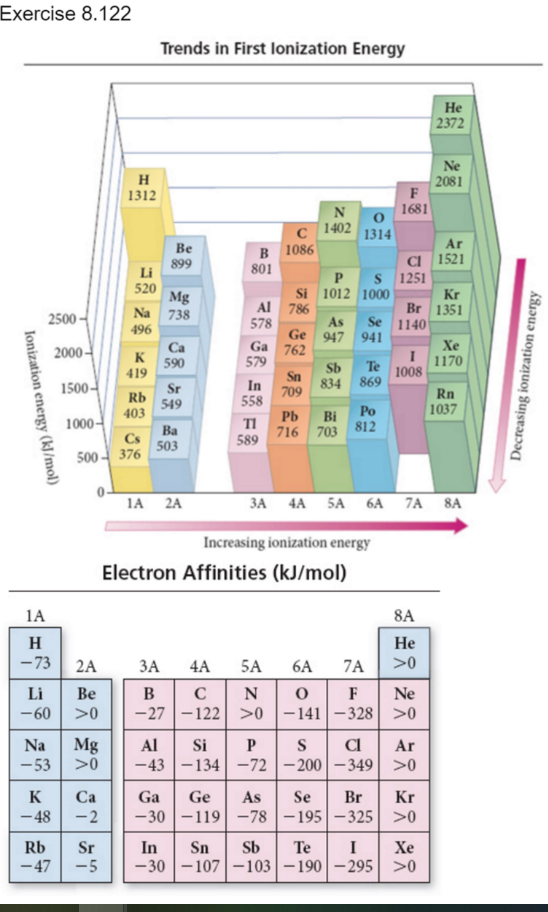# Problem: Using the data in the figures above calculate ΔE for the reaction Na(g)+Cl(g)?Na+(g)+Cl?(g)

###### FREE Expert Solution

To calculate ΔE for the reaction: Na(g)+Cl(g) → Na+(g)+Cl-(g)

First ionization energy → removing the first valence electron from neutral Na

N→ Na+ + e-                 I. E. = 496 kJ/mol

Electron affinity is the energy change from the addition of 1 e to a gaseous element/ion.

Cl(g) + e- Cl-(g)               E. A. = -349 kJ/mol

81% (407 ratings)###### Problem DetailsUsing the data in the figures above calculate ΔE for the reaction Na(g)+Cl(g)?Na+(g)+Cl?(g)